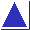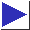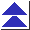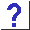# comp.lang.c FAQ list ·Question 17.3

Q: Here's a neat trick for checking whether two strings are equal:

`if(!strcmp(s1, s2))`
Is this good style?

A: It is not particularly good style, although it is a popular idiom. The test succeeds if the two strings are equal, but the use of ! (``not'') suggests that it tests for inequality.

Another option is to define a macro:

```	#define Streq(s1, s2) (strcmp((s1), (s2)) == 0)
```
which you can then use like this:
```	if(Streq(s1, s2))
```

Another option (which borders on preprocessor abuse; see question 10.2) is to define

```	#define StrRel(s1, op, s2) (strcmp(s1, s2) op 0)
```
after which you can say things like
```	if(StrRel(s1, ==, s2)) ...
if(StrRel(s1, !=, s2)) ...
if(StrRel(s1, >=, s2)) ...
```